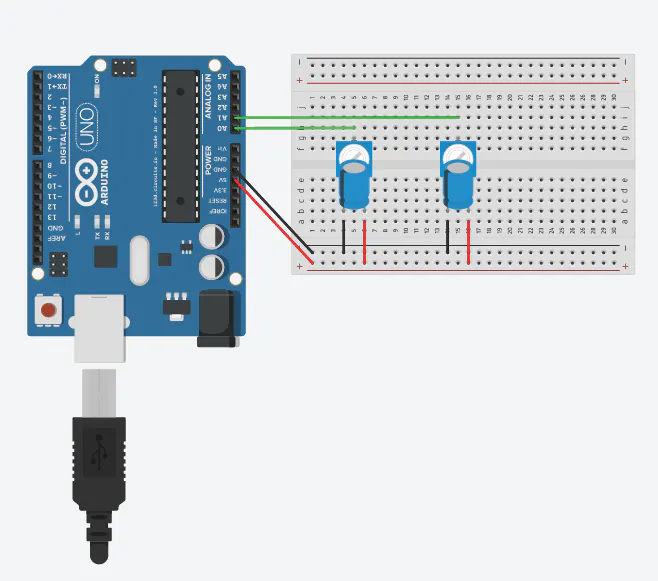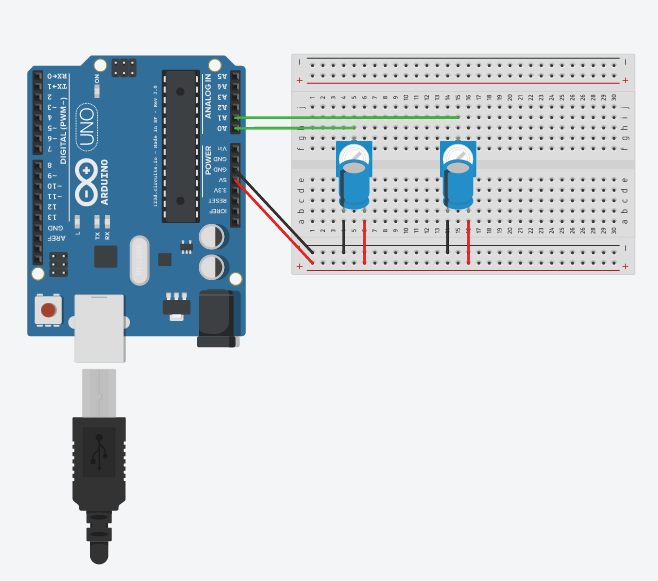Project showcasePlaying a simple Pong game with two potentiometers connected to an Arduino. A Python script on a PC is reading data and displaying the game.

• 3,548 views
• 13 respects

## Components and supplies

This is a two-player game of Pong played by rotating two potentiometers, just like the original! The potentiometers are connected to an Arduino, which sends the values over serial. A Python script is running on the PC that reads the serial data and updates the game. The script uses the Curses library to make printing a game in an x, y coordinate system within a console easier. The potentiometers determine the Y-position within the console and the ball moves automatically based on the Arduino's clock. The ball bounces off of each of the paddles, and the script ends when the ball gets past one of the paddles. When the script ends the winner is reported.

## Code

##### Python codePython
This is the code that runs on the PC. It is written in Python and is responsible for reading serial data from the Arduino and using the Curses library to draw the game on a terminal (size 80x24).
```# Imports
import serial
import curses

# Constants
HEIGHT = 24
WIDTH = 80
BALL_CHAR = '*'
GAME_SPEED = 100

# Classes
class Timer:

def __init__(self, initial_time, target_time):
self.last_reset_time = initial_time
self.target_time = target_time
self.elapsed_time = 0
self.times_reset = 0

def check(self, current_time):
self.elapsed_time = current_time - self.last_reset_time
if self.elapsed_time >= self.target_time:
self.reset(current_time)
return True
else:
return False

def reset(self, current_time):
self.last_reset_time = current_time

# Functions
def map(val, in_min, in_max, out_min, out_max):
return int((val - in_min) * (out_max - out_min) / (in_max - in_min) + out_min)

line = str(raw_line)
line = line[2:] #Remove the unicode characters
line = line[:-5] #Remove the cariage return and newline from the end of the string
data = line.split(" ")
#Convert parsed strings into integers
try:
time = int(data)
p1_pot = int(data)
p2_pot = int(data)
return time, p1_pot, p2_pot, True
except:
return -1, -1, -1, False

game_lost = False
loss_message = "No one has lost"
if update_ball:
#Update ball's position based on the x and y velocities
ball_x = ball_x + dx
ball_y = ball_y + dy
# Check to see if the ball has hit the right wall
if ball_x >= WIDTH - 1:
ball_x = WIDTH - 1
game_lost = True
loss_message = "Player 1 won! Player 2 is pretty sucky though..."
# Check to see if the ball has hit the left wall
if ball_x <= 0:
ball_x = 0
game_lost = True
loss_message = "Player 2 won! Player 1 is pretty sucky though..."
# Check if the ball has bounced off the left paddle
dx = -dx
# Check to see if the ball has bounced off the right paddle
dx = -dx
# Check to see if the ball hit the bottom wall
if ball_y >= (HEIGHT - 1):
ball_y = HEIGHT -1
dy = -dy
# Check to see if the ball hit the top wall
if ball_y <= 0:
ball_y = 0
dy = -dy

# Check to see if the paddle does not pass through the top
# Check to see if the paddles do not pass through the bottom

# Erase old game
screen.clear()
# Draw new ball
screen.refresh()
return ball_x, ball_y, dx, dy, game_lost, loss_message

# Start main program
try:
serial_device = serial.Serial('/dev/ttyACM0', 9600)
except:
print("No serial device found")
exit()

# Initilize timer after serial comminications have reset
initial_time, initial_pot, temp_bool, temp_string = read_serial(serial_device)
# timer_initialized = False
# while not timer_initialized:
#     initial_time, initial_pot, temp_bool, temp_string = read_serial(serial_device)
#     if initial_time == 0:
#         timer_initialized = True
timer = Timer(initial_time, GAME_SPEED)

# Initialize game variables
screen = curses.initscr()
screen.refresh()
ball_x = int(WIDTH / 2)
ball_y = int(HEIGHT / 2)
ball_dx = 3
ball_dy = 2
game_lost = False

while not game_lost:
left_paddle_y = map(p1_pot, 0, 1023, 0, HEIGHT - 1)
right_paddle_y = map(p2_pot, 0, 1023, 0, HEIGHT - 1)
is_time_elapsed = timer.check(time)
# Update game

curses.endwin()
print(loss_message)
```
##### Arduino codeArduino
The sketch running on the Arduino. It is responsible for reading the values of the two potentiometers and send them serially.
```int player1_val;
int player2_val;
const int player1_pot = A0;
const int player2_pot = A1;

void setup() {
pinMode(player1_pot, INPUT);
pinMode(player2_pot, INPUT);
Serial.begin(9600);
}

void loop() {

Serial.print(millis());
Serial.print(" ");
Serial.print(player1_val);
Serial.print(" ");
Serial.println(player2_val);

}
```

## Schematics#### Published on

December 18, 2015

#### Members who respect this project

See similar projects
you might like

#### RING PONG

Project showcase by aerodynamics

• 7,675 views
• 15 respects

#### ARDUINO PONG

Project tutorial by raul

• 2,648 views
• 1 comment
• 8 respects

#### Arduino Serial Plotter & Capacitors

Project in progress by Koiotti

• 2,128 views
• 5 respects

#### How to make Arduino Pong Game-Arduino Project

Project tutorial by Mrmodder

• 5,532 views
• 1 comment
• 4 respects

#### Ponguino Arduino Pong Game

Project tutorial by 5vdc

• 1,013 views